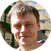Skip to main content

# Statistical models in population geneticsPublished on March 31, 2016   35 min
• Add to
• Review

#### Other Talks in the Series: Statistical Genetics

0:00
ASGER HOBOLTH: Hello everybody, my name is Asger Hobolth, and I will give you an introduction to statistical models in population genetics.
0:09
So the main purpose of statistical models in population genetics is to formulate models that allows us to understand and describe genetic variation observed in DNA sequences. I've decided to divide my talk into two parts, responding to do different summaries of genetic variation from DNA sequence data. So in the first part of my talk, I will talk about the site frequency spectrum, which is an often-used statistic for summarizing genetic variation. And in the second part of my talk, I will talk about how you can model distances between heterozygote sites. This is a more involved problem, and the theory's a bit more difficult. But this is also an often-used statistic for summarizing genetic variation. The theory that we will need is the so-called Wright-Fisher model, which is a forward model of evolution. And then it is the corresponding backwards process, which is called the coalescent process. And this coalescent process basically gives us what is called a tree, and we will have to add mutations on this tree. And this will give us a model for DNA sequences. And basically, using coalescent process with mutations, we can derive the side frequency spectrum. So we can derive the summary of what we expect the first summary statistics to look like. And in the second part of the talk, we will have to add the so-called recombination process through the basic coalescent process, and we will have to, instead of work with the trees, we will have to work with the so-called ancestral recombination graph. But I will come back to all this.
Hide